International
Tables for
Crystallography
Volume E
Subperiodic groups
Edited by V. Kopský and D. B. Litvin

International Tables for Crystallography (2006). Vol. E, ch. 1.2, pp. 14-15   | 1 | 2 |

## Section 1.2.8. Asymmetric unit

V. Kopskýa and D. B. Litvinb*

aDepartment of Physics, University of the South Pacific, Suva, Fiji, and Institute of Physics, The Academy of Sciences of the Czech Republic, Na Slovance 2, PO Box 24, 180 40 Prague 8, Czech Republic, and bDepartment of Physics, Penn State Berks Campus, The Pennsylvania State University, PO Box 7009, Reading, PA 19610-6009, USA
Correspondence e-mail:  u3c@psu.edu

### 1.2.8. Asymmetric unit

| top | pdf |

An asymmetric unit of a subperiodic group is a simply connected smallest part of space from which, by application of all symmetry operations of the subperiodic group, the whole space is filled exactly. For three-dimensional (two-dimensional) space groups, because they contain three-dimensional (two-dimensional) translational symmetry, the asymmetric unit is a finite part of space [see Section 2.2.8of IT A (2005)]. For subperiodic groups, because the translational symmetry is of a lower dimension than that of the space, the asymmetric unit is infinite in size. We define the asymmetric unit for subperiodic groups by setting the limits on the coordinates of points contained in the asymmetric unit.

#### 1.2.8.1. Frieze groups

| top | pdf |

For all frieze groups, a limit is set on the x coordinate of the asymmetric unit by the inequality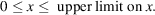For the y coordinate, either there is no limit and nothing further is written, or there is the lower limit of zero, i.e.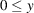.

#### Example: The frieze group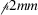(F6)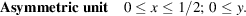#### 1.2.8.2. Rod groups

| top | pdf |

For all rod groups, a limit is set on the z coordinate of the asymmetric unit by the inequality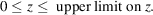For each of the x and y coordinates, either there is no limit and nothing further is written, or there is the lower limit of zero.

For tetragonal, trigonal and hexagonal rod groups, additional limits are required to define the asymmetric unit. These limits are given by additional inequalities, such as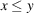and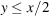. Fig. 1.2.8.1schematically shows the boundaries represented by such inequalities.Figure 1.2.8.1 | top | pdf |Boundaries used to define the asymmetric unit for (a) tetragonal rod groups and (b) trigonal and hexagonal rod groups.

#### Example: The rod group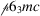(R70)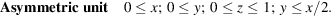#### 1.2.8.3. Layer groups

| top | pdf |

For all layer groups, limits are set on the x coordinate and y coordinate of the asymmetric unit by the inequalities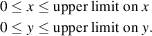For the z coordinate, either there is no limit and nothing further is written, or there is the lower limit of zero.

For tetragonal/square, trigonal/hexagonal and hexagonal/hexagonal layer groups, additional limits are required to define the asymmetric unit. These additional limits are given by additional inequalities. Fig. 1.2.8.2schematically shows the boundaries represented by these inequalities. For trigonal/hexagonal and hexagonal/hexagonal layer groups, because of the complicated shape of the asymmetric unit, the coordinates (x, y) of the vertices of the asymmetric unit with theplane are given.Figure 1.2.8.2 | top | pdf |Boundaries used to define the asymmetric unit for (a) tetragonal/square layer groups and (b) trigonal/hexagonal and hexagonal/hexagonal layer groups. In (b), the coordinates (x, y) of the vertices of the asymmetric unit with theplane are also given.

#### Example: The layer group p3m1 (L69)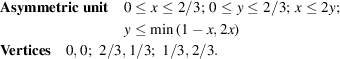### References

International Tables for Crystallography (2005). Vol. A. Space-group symmetry, edited by Th. Hahn. Heidelberg: Springer. [Previous editions: 1983, 1987, 1992, 1995 and 2002. Abbreviated as IT A (2005).]